SQL Server Matrix Workbench

In this workbench, Robyn Page and Phil Factor decide to tackle the subject of Matrix handling and Matrix Mathematics in SQL. They maintain that 'One just needs a clear head and think in terms of set-based operations'

I think that we need to lay to rest the idea that you cannot do matrix operations in SQL. They are just as easy as they are in a procedural language. One just needs a clear head and think in terms of set-based operations.

If you’re not aware of the usefulness of matrix operations, then you’ll be surprised. They underlie a lot of linear equations and statistics (We do a cool Orthogonal Factor Analysis in SQL!). They’re used a lot in games programming, and bitmap manipulation. We tend to use them for sticky SQL problems such as reserving seats or timetabling. Once you’ve started, you’ll find a lot of SQL problems that can be solved with matrices. (Just in case the formatting goes awry, we’re including the SQL Source file in the downloads at the bottom of the article.) Please remember that this isn’t production-quality code: as in all of our workbenches, we’re keeping things simple to illustrate the points as clearly as we can.

We’ll create a table to store two-dimensional arrays that contain an exact number with two digits precision. You’ll see that we can create any number of matrixes in this array, which cuts down on the chore of creating tables. It also means that functions and procedures can be hardwired to a common matrix table. Of course, you would create the ‘element’ type according to your data.

Yes, this allows the use of sparse arrays, but you would usually need to handle gaps. Note the compound primary key. This automatically prevents duplication of data in a cell (try it in order to convice yourself) and provides a reasonable index for most operations. If you use very large matrixes, you will need to provide an extra covering index.

Now let’s fill matrix ‘A’ with some spoof data so we can just do some simple stuff such as summing the rows and columns.

We’ll choose a ten by fifteen matrix:

Now the simple way to do a summation is by using the built-in grouping functions such as `ROLLUP` and `CUBE`: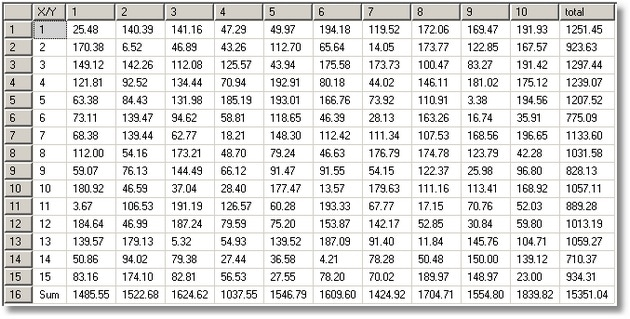Note that, if you are using sparse arrays, you may need to do this.

First, we’ll put in a few drips and drabs of data.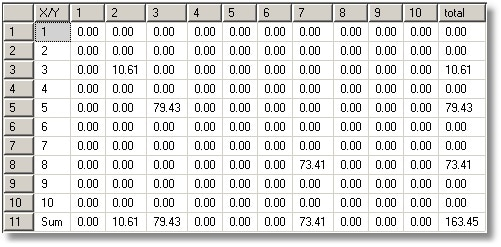If this is tedious, then use a procedure. Well, of course it’s tedious!

And while we’re about it, we’ll add a procedure to do a simple matrix. This will just be a cut-down version of the one above.

Okay, let’s try them out!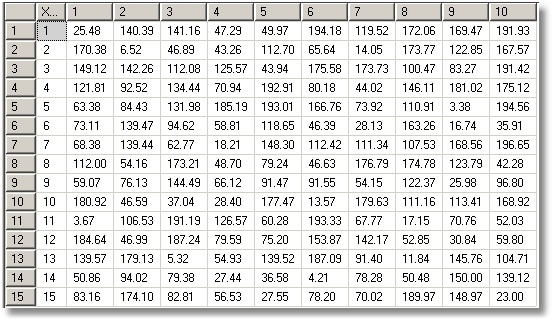In order to do a few common matrix examples, we need to devise a few helper functions to allow us to get matrix data in there easily, in the format that is easy to check for errors. This is very handy when you are doing regression testing.

Before we do the routine, let’s just make sure you have a number table…

Now we can add the function that takes a string representation of a matrix, just like you see in the textbooks, and returns the SQL equivalent (please try it out just to see what it does). It could be done iteratively, but we have opted for the must faster TSQL-Special technique. If you are using a different dialect, you’ll have to do it the slow iterative way instead, but we’re using SQL Server!

So let’s test it out now….

Wee!! that worked, didn’t it!

Let’s double-check…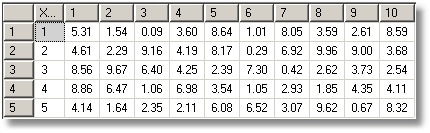Now we can get started!

Matrix multiplication by a scalar

And let’s see the result….

So scalar arithmetic is absurdly simple and need concern us no longer.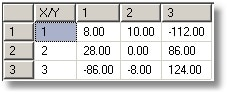Here is how we do Matrix Addition.

And let’s see the result….

Matrix subtraction

And matrix subtraction is just as easy.

And now let’s see it!

Matrix Multiplication

So you think you can do the same thing with matrix multiplication eh? Just use a ‘*’ instead of a ‘-‘? Think again. this is where it gets more complicated.
We’ll multiply two nice simple matrices…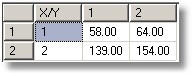Matrix Horizontal concatenation

Horizontal concatenation is cool.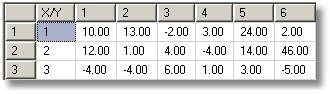Matrix Vertical Concatenation

Or we can do it vertically (sentiment about the variable applies here too).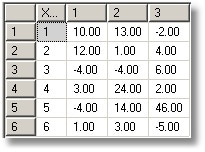Matrix Transposition

Fine, so how do we do Matrix transposition?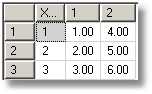Rendering a matrix as a string

So now, just to finish off with a flourish, we’ll reverse-out our `MatrixValuesOf` Function, so we can represent the results in a string. This means that we could make an easier test harness and could squirrel matrixes away in string form.

So, what goes in comes out (it took a little bit of heaving and grunting). So this is as far as we’ll take it in this workbench and we’ll leave you to figure out a few little puzzles such as inversion and determinants. Now, the next puzzle is how one can use these techniques to solve other programming puzzles. It would be fascinating to learn how this sort of technique can be used.